# Primitive Polynomials

Primitive polynomials for Galois field generation up to GF(2^1200), GF(3^660), GF(5^430), and GF(7^358)

## Examples

### Basic Examples

Retrieve the resource:

 In:=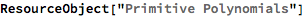Out=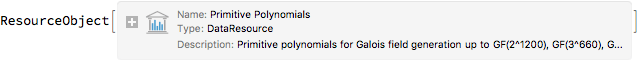Retrieve the default content:

 In:=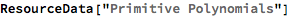Out=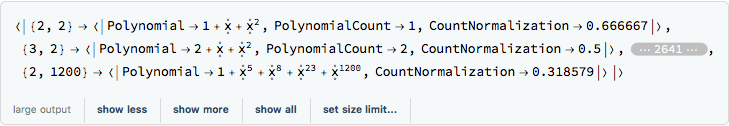### Visual Examples

Show the data for GF(267). The number 267-1 has a factorization 193707721×761838257287 (Cole, 1903) that gives a normalization close to 1:

 In:=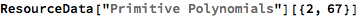Out=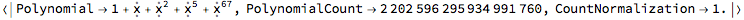Generate the first 600 powers of x using a polynomial modulus of 1+x+x2+x5+x67:

 In:=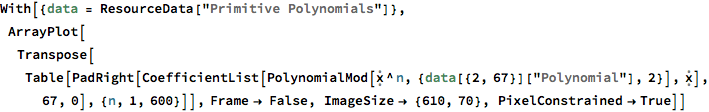Out=Show the approximate count via multiplication of the count normalization by (pn-1)/n and compare that to the actual polynomial count:

 In:=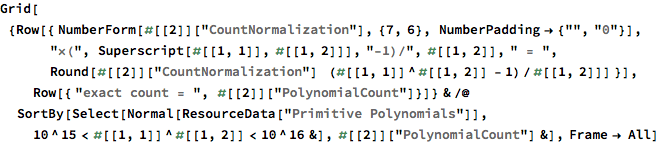Out=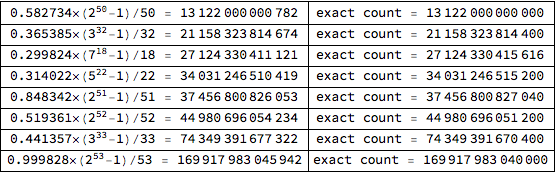Show the count normalizations for the first four primes:

 In:=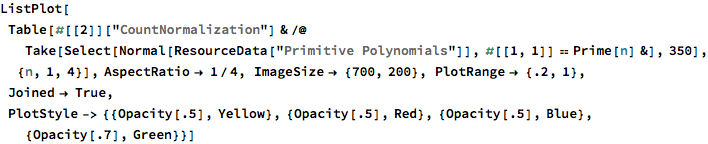Out=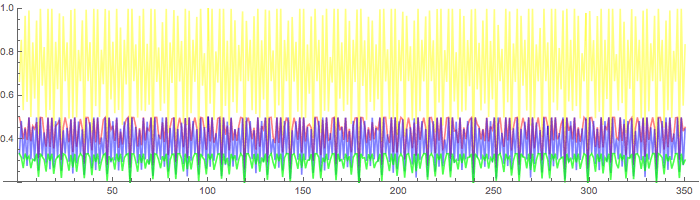Show the degrees where trinomial primitive polynomials exist for the first four primes:

 In:=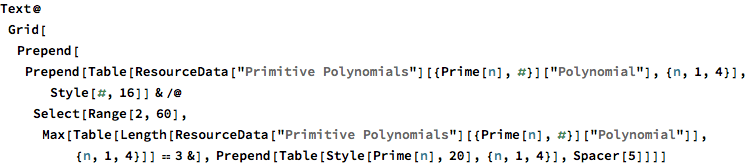Out=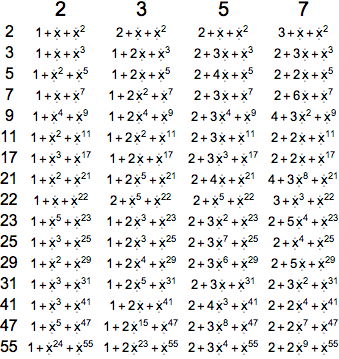Wolfram Research, "Primitive Polynomials" from the Wolfram Data Repository (2017) https://doi.org/10.24097/wolfram.48521.data